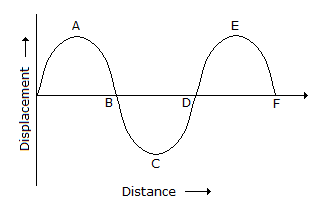# Civil Engineering - Elements of Remote Sensing

### Exercise :: Elements of Remote Sensing - Section 1

11.

Which one of the following residual biases involves the GPS accuracy ?

 A. Satellite dependent baises due to uncertainty in the orbital parameters of the satellite. B. Receiver-depandent biases due to clock stability with line C. Signal propagation baises due to the sphere and troposphere propogation. D. All the above

Explanation:

No answer description available for this question. Let us discuss.

12.

Pick up the correct definition from the following with response to GIS.

 A. Common boundry between two areas of a locality is known as adjacency. B. The area features which are wholly contained within another area feature, is known so containment. C. The geometric property which describes the linkage between line features is defined as connectivity. D. All of these.

Explanation:

No answer description available for this question. Let us discuss.

13.

Which one of the following errors is produced by platform characteristics of the sensor ?

 A. altitude variation B. altitude C. orbit drift D. All of these

Explanation:

No answer description available for this question. Let us discuss.

14.

The coherence length over which there is a strong relationship between amplitudes is;

 A. directly proportional to the bandwidth B. inversely proportional to the bandwidth C. the square of the bandwidth D. none of these

Explanation:

No answer description available for this question. Let us discuss.

15.

In the given figure the phase ofA. crest A is π/2 B. B crossing is π C. trough C is 3 π/2 D. All of these